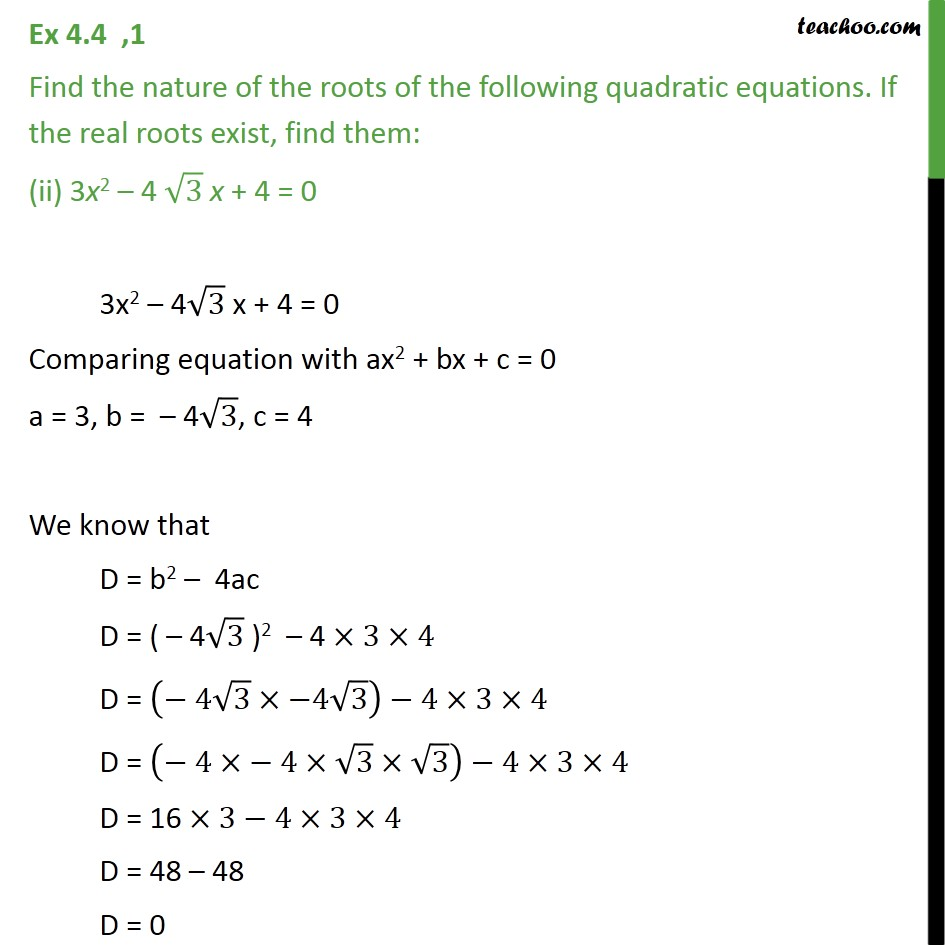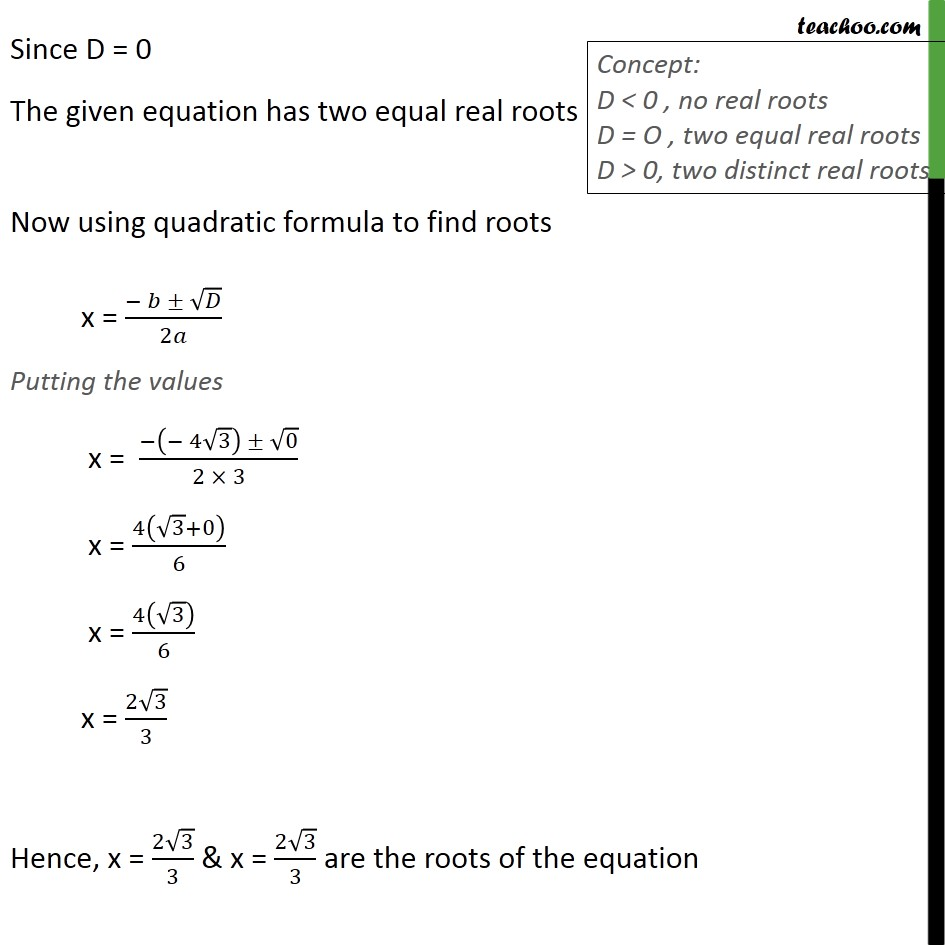Completing the square and Word Problems

Chapter 4 Class 10 Quadratic Equations
Serial order wiseLearn in your speed, with individual attention - Teachoo Maths 1-on-1 Class

### Transcript

Ex 4.3 ,1 Find the nature of the roots of the following quadratic equations. If the real roots exist, find them: (ii) 3x2 – 4 √3 x + 4 = 0 3x2 – 4√3 x + 4 = 0 Comparing equation with ax2 + bx + c = 0 a = 3, b = – 4√3, c = 4 We know that D = b2 – 4ac D = ( – 4√3 )2 – 4 ×3×4 D = (− 4√3×−4√3)−4×3×4 D = (− 4×− 4×√3×√3)−4×3×4 D = 16 ×3−4×3×4 D = 48 – 48 D = 0 Since D = 0 The given equation has two equal real roots Now using quadratic formula to find roots x = (− 𝑏 ± √𝐷)/2𝑎 Putting the values x = (−(− 4√3) ± √0)/(2 × 3) x = 4(√3+0)/6 x = 4(√3)/6 x = (2√3)/3 Hence, x = (2√3)/3 & x = (2√3)/3 are the roots of the equation